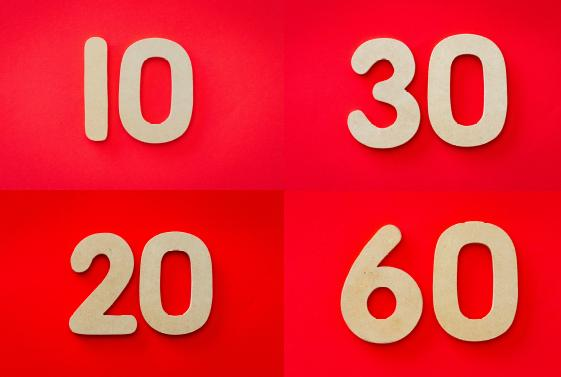Let’s declare our function:

For starters, we’ve named our function, and we’ve specified the expected parameters. If we call the `randomNumFromRange` function now, we’d get the passed-in arguments’ values logged out to the console.

Now we’ll use `Math.random()`.

This will give us any range from inclusive `0` to exclusive `1`. But how do we do it for a range of, for example, `30` to `60`?

To solve this issue, we need to think about our maximum number in the range, which is `60`. How do we get it?

With this, we’ll get any number between `1` and `60`. To prove it, let’s look at the lowest possible result of `Math.random()`:

Obviously, the result of the above will always be `1`. How about using the largest possible result of `Math.random()`?

The result of `Math.floor(0.99 * 60)` is `59`, and with a `+ 1`, we get `60`.

So, we’re indeed never going to get the result over `60`. Now let’s look at our code so far and think about how to get the result of not less than `30`.

To do that, let’s begin with replacing the `Math.floor()` with `Math.ceil()`, and a `+ 1` with a `+ 0`.

Now we need to get a range, using:

This will give us a range of 30 numbers, starting anywhere from `0` to `30`.

To move the range of `0...30` to `30...60`, we need to move the “slider” by `30` numbers up; this is the exact value of our `minNum`.

This results in the following code:

Now we can remove magic numbers and use parameters instead:

You can test it out by calling it like, for example, this:

It’s good to test it this way because you can expect to get only 2 numbers, either a `31` or a `32`.

We can further simplify our code like this:

To reiterate, in the above code, `maxNum - minNum` sets up the range, and `+ minNum` moves it’s starting point from zero to the value of `minNum`.

Finally, we can re-write this code as an ES6 function:

Note:
This exercise comes from Book 3 of my book series on JS, available on Leanpub.com.